# 题目描述（中等难度）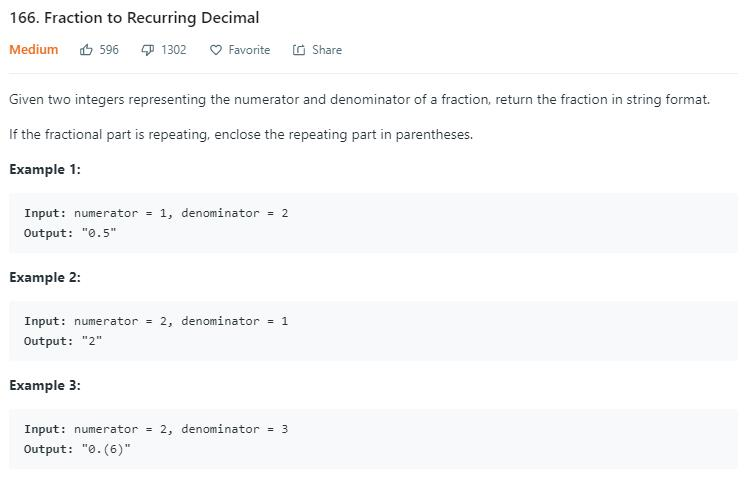# 解法一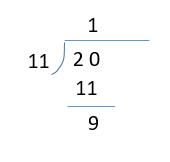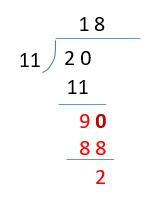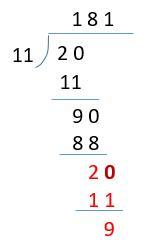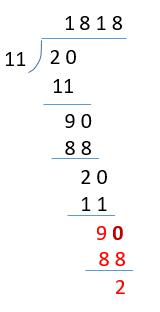• 第一种情况，余数为 0，说明没有循环小数。

• 第二种情况，一开始这里爬坑了。开始觉得只要商里边出现重复的数字（不考虑整数部分的数字，也就是上边例子的第一个 1），就可以认为出现了循环小数。

比如上边的例子，8 第二次出现，所以到这里不再计算。而循环小数部分就是和当前数字重复的位置到当前位置的前一个，也就是 81。所以最终结果就是 1.(81)

但提交的时候，出现了一个反例，如下图。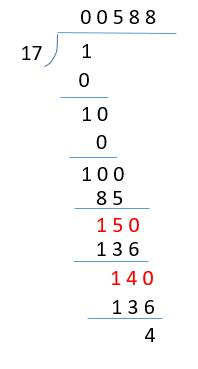虽然出现了重复的 8，但最终结果并不是 8 循环。很明显下次是 40 / 17，需要商 2。至于原因就是两次商 8 所对应的被除数并不一样，第一次是 150 ，第二次是 140

所以为了判断是否出现循环小数，我们不应该判断是否出现了重复的商，而是应该判断是否出现了重复的被除数。

public String fractionToDecimal(int numerator, int denominator) {
long num = numerator;
long den = denominator;
String sign = "";
//确定符号
if (num > 0 && den < 0 || num < 0 && den > 0) {
sign = "-";
}
//转为正数
num = Math.abs(num);
den = Math.abs(den);
//记录整数部分
long integer = num / den;
//计算余数
num = num - integer * den;
HashMap<Long, Integer> map = new HashMap<>();
int index = 0;
String decimal = "";//记录小数部分
int repeatIndex = -1;//保存重复的位置
while (num != 0) {
num *= 10;//余数乘以 10 作为新的被除数
if (map.containsKey(num)) {
repeatIndex = map.get(num);
break;
}
//保存被除数
map.put(num, index);
//保存当前的商
long decimalPlace = num / den;
//加到所有的商中
decimal = decimal + decimalPlace;
//计算新的余数
num = num - decimalPlace * den;
index++;
}
//是否存在循环小数
if (repeatIndex != -1) {
String dec = decimal;
return sign + integer + "." + dec.substring(0, repeatIndex) + "(" + dec.substring(repeatIndex) + ")";
} else {
if (decimal == "") {
return sign + integer;
} else {
return sign + integer + "." + decimal;
}
}
}